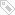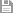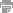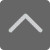# 六年级数学知识点总结

2018-07-11 10:50:02 　来源：网络整理

六年级数学知识点总结同学们知道学习的重要性，大家在学习中，首先要掌握知识点，其次学习方法也很重要。关于小学各科的知识点总结有哪些内容呢？下面是小编为大家整理的六年级数学知识点总结，希望对大家有所帮助。

常用的数量关系式

1、每份数×份数=总数 总数÷每份数=份数 总数÷份数=每份数

2、1倍数×倍数=几倍数 几倍数÷1倍数=倍数 几倍数÷倍数=1倍数

3、速度×时间=路程 路程÷速度=时间 路程÷时间=速度

4、单价×数量=总价 总价÷单价=数量 总价÷数量=单价

5、工作效率×工作时间=工作总量 工作总量÷工作效率=工作时间

工作总量÷工作时间=工作效率

6、加数+加数=和 和-一个加数=另一个加数

7、被减数-减数=差 被减数-差=减数 差+减数=被减数

8、因数×因数=积 积÷一个因数=另一个因数

9、被除数÷除数=商 被除数÷商=除数 商×除数=被除数

小学数学图形公式

1、正方形 (C：周长 S：面积 a：边长)

周长=边长×4 C=4a 面积=边长×边长 S=a×a

2、正方体 (V:体积 a:棱长 )

表面积=棱长×棱长×6 S表=a×a×6

体积=棱长×棱长×棱长 V=a×a×a

3、长方形( C：周长 S：面积 a：边长)

周长=(长+宽)×2 C=2(a+b)

面积=长×宽 S=ab

4、长方体 (V:体积 s:面积 a:长 b: 宽 h:高)

(1)表面积(长×宽+长×高+宽×高)×2 S=2(ab+ah+bh)

(2)体积=长×宽×高 V=abh

5、三角形 (s：面积 a：底 h：高)

面积=底×高÷2 s=ah÷2

三角形高=面积 ×2÷底 三角形底=面积 ×2÷高

6、平行四边形 (s：面积 a：底 h：高)

面积=底×高 s=ah

7、梯形 (s：面积 a：上底 b：下底 h：高)

面积=(上底+下底)×高÷2 s=(a+b)× h÷2

8、圆形 (S：面积 C：周长 л d=直径 r=半径)

(1)周长=直径×л=2×л×半径 C=лd=2лr

(2)面积=半径×半径×л

9、圆柱体 (v:体积 h:高 s：底面积 r:底面半径 c:底面周长)

(1)侧面积=底面周长×高=ch(2лr或лd) (2)表面积=侧面积+底面积×2

(3)体积=底面积×高 (4)体积=侧面积÷2×半径

圆锥体 (v:体积 h:高 s：底面积 r:底面半径)

体积=底面积×高÷3

11、总数÷总份数=平均数

12、和差问题的公式

(和+差)÷2=大数 (和-差)÷2=小数

13、和倍问题

和÷(倍数-1)=小数 小数×倍数=大数 (或者 和-小数=大数)

14、差倍问题

差÷(倍数-1)=小数 小数×倍数=大数 (或 小数+差=大数)

15、相遇问题

相遇路程=速度和×相遇时间

相遇时间=相遇路程÷速度和

速度和=相遇路程÷相遇时间

16、浓度问题

溶质的重量+溶剂的重量=溶液的重量

溶质的重量÷溶液的重量×100%=浓度

溶液的重量×浓度=溶质的重量

溶质的重量÷浓度=溶液的重量

17、利润与折扣问题

利润=售出价-成本

利润率=利润÷成本×100%=(售出价÷成本-1)×100%

涨跌金额=本金×涨跌百分比

利息=本金×利率×时间

以上就是小编为大家整理的六年级数学知识点总结，同学们还有其他学习上的问题，可拨打爱智康课程免费咨询热线电话：4000-121-121 .那里有专业的老师为大家解答。标签：　六年级数学知识点总结保存　｜打印　｜关闭